# Mathematical Logic Ex 1.2 - Maharashtra Board 12th Maths Solutions Chapter 1

### Question 1.Construct the truth table for each of the following statement patterns:

(i) [(p → q) ∧ q] → p
Solution :
Here are two statements and three connectives.
∴ there are 2 × 2 = 4 rows and 2 + 3 = 5 columns in the truth table.

(ii) (p ∧ ~q) ↔ (p → q)
Solution: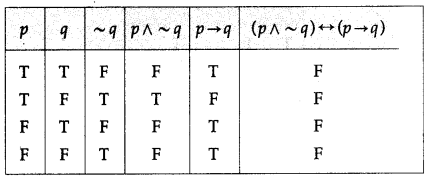(iii) (p ∧ q) ↔ (q ∨ r)
Solution: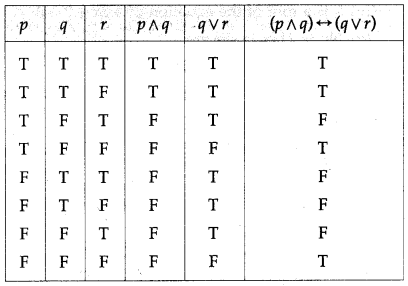(iv) p → [~(q ∧ r)]
Solution:

(v) ~p ∧ [(p ∨ ~q ) ∧ q]
Solution: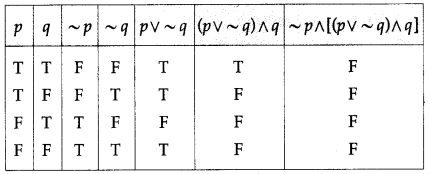(vi) (~p → ~q) ∧ (~q → ~p)
Solution: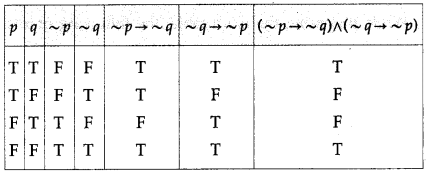(vii) (q → p) ∨ (~p ↔ q)
Solution:

(viii) [p → (q → r)] ↔ [(p ∧ q) → r]
Solution: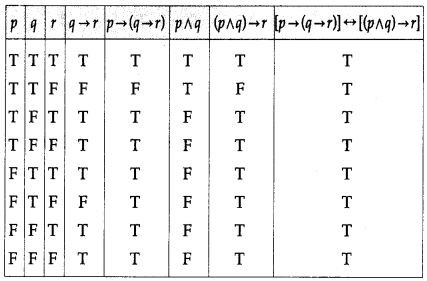(ix) p → [~(q ∧ r)]
Solution:

(x) (p ∨ ~q) → (r ∧ p)
Solution: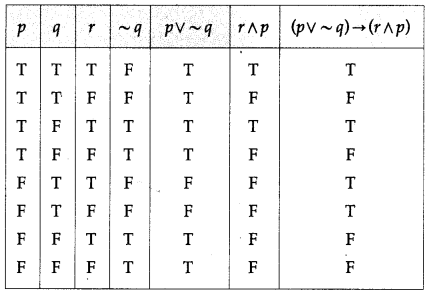### Question 2.Using truth tables prove the following logical equivalences.

(i) ~p ∧ q ≡ (p ∨ q) ∧ ~p
Solution: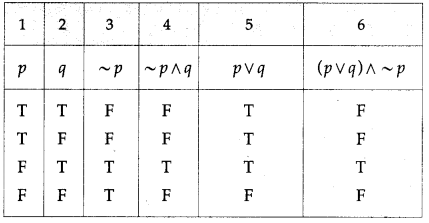The entries in the columns 4 and 6 are identical.
∴ ~p ∧ q ≡ (p ∨ q) ∧ ~p.

(ii) ~(p ∨ q) ∨ (~p ∧ q) ≡ ~p
Solution:
The entries in the columns 3 and 7 are identical.
∴ ~(p ∨ q) ∧ (~p ∧ q) = ~p.

(iii) p ↔ q ≡ ~[(p ∨ q) ∧ ~(p ∧ q)]
Solution: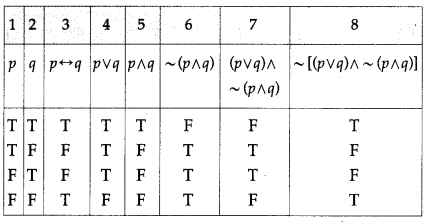The entries in the columns 3 and 8 are identical.
∴ p ↔ q ≡ ~[(p ∨ q) ∧ ~(p ∧ q)].

(iv) p → (q → p) ≡ ~p → (p → q)
Solution: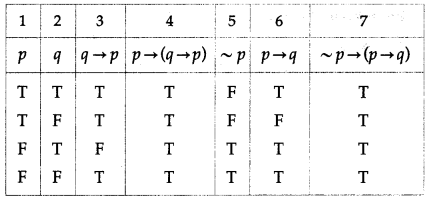The entries in the columns 4 and 7 are identical.
∴ p → (q → p) ≡ ~p → (p → q).

(v) (p ∨ q ) → r ≡ (p → r) ∧ (q → r)
Solution:
The entries in the columns 5 and 8 are identical.
∴ (p ∨ q ) → r ≡ (p → r) ∧ (q → r).

(vi) p → (q ∧ r) ≡ (p → q) ∧ (p → r)
Solution: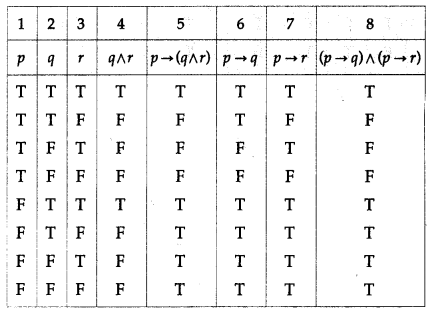The entries in the columns 5 and 8 are identical.
∴ p → (q ∧ r) ≡ (p → q) ∧ (p → r).

(vii) p ∧ (q ∨ r) ≡ (p ∧ q) ∨ (p ∧ r)
Solution: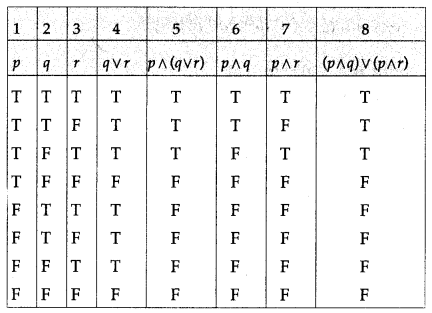The entries in the columns 5 and 8 are identical.
∴ p ∧ (q ∨ r) ≡ (p ∧ q) ∨ (p ∧ r).

(viii) [~(p ∨ q) ∨ (p ∨ q)] ∧ r ≡ r
Solution:
The entries in the columns 3 and 7 are identical.
∴ [~(p ∨ q) ∨ (p ∨ q)] ∧ r ≡ r.

(ix) ~(p ↔ q) ≡ (p ∧ ~q) ∨ (q ∧ ~p)
Solution: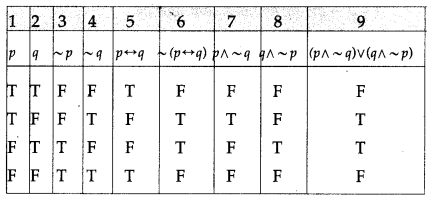The entries in the columns 6 and 9 are identical.
∴ ~(p ↔ q) ≡ (p ∧ ~q) ∨ (q ∧ ~p).

### Question 3.Examine whether each of the following statement patterns is a tautology or a contradiction or a contingency.

(i) (p ∧ q) → (q ∨ p)
Solution: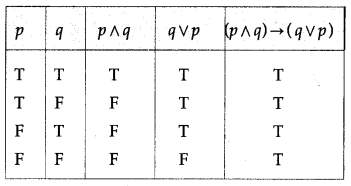All the entries in the last column of the above truth table are T.
∴ (p ∧ q) → (q ∨ p) is a tautology.

(ii) (p → q) ↔ (~p ∨ q)
Solution:
All the entries in the last column of the above truth table are T.
∴ (p → q) ↔ (~p ∨ q) p is a tautology.

(iii) [~(~p ∧ ~q)] ∨ q
Solution: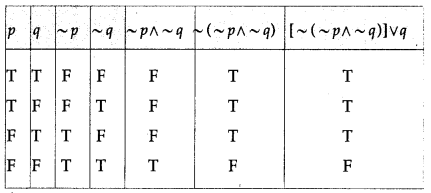The entries in the last column of the above truth table are neither all T nor all F.
∴ [~(~p ∧ ~q)] ∨ q is a contingency.

(iv) [(p → q) ∧ q)] → p
Solution: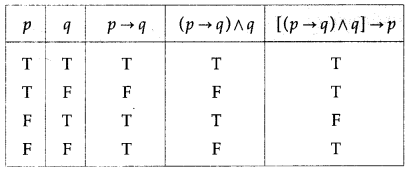The entries in the last column of the above truth table are neither all T nor all F.
∴ [(p → q) ∧ q)] → p is a contingency

(v) [(p → q) ∧ ~q] → ~p
Solution:
All the entries in the last column of the above truth table are T.
∴ [(p → q) ∧ ~q] → ~p is a tautology.

(vi) (p ↔ q) ∧ (p → ~q)
Solution: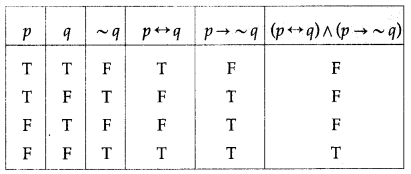The entries in the last column of the above truth table are neither all T nor all F.
∴ (p ↔ q) ∧ (p → ~q) is a contingency.

(vii) ~(~q ∧ p) ∧ q
Solution:

The entries in the last column of the above truth table are neither all T nor all F.
∴ ~(~q ∧ p) ∧ q is a contingency.

(viii) (p ∧ ~q) ↔ (p → q)
Solution: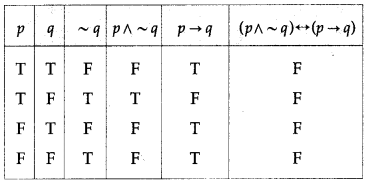All the entries in the last column of the above truth table are F.
∴ (p ∧ ~q) ↔ (p → q) is a contradiction.

(ix) (~p → q) ∧ (p ∧ r)
Solution:
The entries in the last column of the above truth table are neither all T nor all F.
∴ (~p → q) ∧ (p ∧ r) is a contingency.

(x) [p → (~q ∨ r)] ↔ ~[p → (q → r)]
Solution: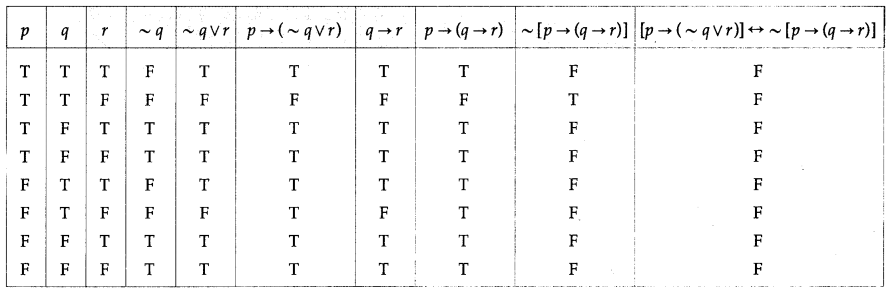All the entries in the last column of the above truth table are F.
∴ [p → (~q ∨ r)] ↔ ~[p → (q → r)] is a contradiction

### 1.2 STATEMENT PATTERN, LOGICAL EQUIVALENCE, TAUTOLOGY, CONTRADICTION, CONTINGENCY.

1.2.1 Statement Pattern :
Letters used to denote statements are called statement letters. Proper combination of statement letters and connectives is called a statement pattern. Statement pattern is also called as a proposition. p → q, p ∧ q, ~ p ∨ q are statement patterns. p and q are their prime components.

A table which shows the possible truth values of a statement pattern obtained by considering all possible combinations of truth values of its prime components is called the truth table of the statement pattern.

1.2.2. Logical Equivalence :
Two statement patterns are said to be equivalent if their truth tables are identical. If statement patterns A and B are equivalent, we write it as A ≡ B.

1.2.3 Tautology, Contradiction and Contingency :
Tautology : A statement pattern whose truth value is true for all possible combinations of truth values of its prime components is called a tautology. We denote tautology by t.  Statement pattern p ∨ ~ p is a tautology.

Contradiction : A statement pattern whose truth value is false for all possible combinations of truth values of its prime components is called a contradiction. We denote contradiction by c. Statement pattern p ∧ ~ p is a contradiction.

Contingency : A statement pattern which is neither a tautology nor a contradiction is called a contingency. p ∧ q is a contingency.

### 1.2 STATEMENT PATTERN, LOGICAL EQUIVALENCE, TAUTOLOGY, CONTRADICTION, CONTINGENCY.

• mathematical logic class 1.2 exercise 1.6 solutions
• mathematical logic exercise 1.2
• mathematical logic exercise 1.3
• mathematical logic exercise 1.2 solutions
• mathematical logic exercise 1.1
• mathematical logic exercise 1.5
• mathematical logic class 12 exercise 1.2
• mathematical logic class 12 exercise 1.2 solutions
• mathematical logic exercise 1.2 new syllabus
• mathematical logic class 1.2 exercise 1.3 solutions
• mathematical logic class 12 exercise 1.3 solutions
• Mathematical Logic - Maharashtra Board 12th Maths Solutions Chapter 1 Ex 1.2